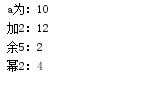• C语言提供了两种非常有用的运算符就是增一和减一运算符 增一运算符是用两个加号来表示的 增一运算的作用使参与运算的值增加一个单位 增一和减一运算符都是一元...而赋值运算的左值不允许是表达式的，因此增一和减一它的
• //赋值运算符，他的优先级低于+—*% int main(){ int a=2; int b=3; a *= b+4; printf("a=%d\n",a);//a=a*(b+4) } int main1() { int a, b, c, d, e; a=b=c=d=e=5; printf("a=%d b=%d c=%d d=%d e=%d\n",a,b...
#include <stdio.h>
//赋值运算符，他的优先级低于+—*%
int main(){
int a=2;
int b=3;
a *= b+4;
printf("a=%d\n",a);//a=a*(b+4)
}

int main1()
{
int a, b, c, d, e;
a=b=c=d=e=5;
printf("a=%d b=%d c=%d d=%d e=%d\n",a,b,c,d,e);
//任何表达式都是有值的
c=(a=b)+(b=c);
printf("%d\n",c);
printf("%d",a>b);//作为一个表达式它的值是0
a++;//单目运算符
a+b;//双目
a>b?c:d;//三目
return 0;
}


展开全文c语言
• ++运算符与赋值运算 简单介绍++运算符 ++和-- 都是运算符，使用的场景还有单独使用和组合使用的区别； 单独使用的情况 单独使用的时候 ++ 就是自增1，–就是自减1； 例如： int a = 1; a++; 这样就是把a的值自增了1...
++运算符与赋值运算
简单介绍++运算符
++和-- 都是运算符，使用的场景还有单独使用和与赋值操作组合使用的区别；
单独使用的情况
单独使用的时候 ++ 就是自增1，–就是自减1； 例如：
int a = 1;
a++;

这样就是把a的值自增了1，a的值就变成了2； 他就相当于 a=a+1;但是他又不完全是。 例如：
byte a =1;
//a=a+1;//会报错
a++;//不会报错

为什么呢？ 简单的赋值操作 就会报错，是因为在赋值操作之前a+1的结果已经变成了int类型，int类型赋值给byte类型是需要强制类型转换的。 然而 ++运算符默认了类型转换，所以不会报错；
与赋值操作组合使用的情况
先上代码：
int a = 1;
int b = a++;
int c = ++a;
System.out.println(a);//3
System.out.println(b);//1
System.out.println(c);//3

这个学了基础的都记住了一规律， ++在前先计算后赋值，++在后先赋值后计算。 所以稍微分析一下： int a =1;此时a的值为1；
int b =  a++;

口诀理解就是 ++在后，先将a的值赋值给了b，所以现在b=1，后进行++运算 a的值自增1变成了2； 记录：a=2;b=1;
int c = ++a;

继续，++在前，先计算++ a自增1，此时a已经变成了3然后赋值给了c 所以c的最终结果是3。 记录：a=3；b=1;c=3 和最后的输出结果是一致的，但是真的是这样的吗？
重点来了！
看++在后的情况
int num=10;
System.out.println(num);//10
num=num++;
System.out.println(num);//10

很奇怪怎么会是10呢？为什么不是11呢？ 用原来的思路分析：++在后先赋值将10赋值给了num，然后进行++运算num自增1应该变成了11才对呀，怎么输出的结果还是10呢？ 其实并不是这样的！ 赋值操作其实是将等号右边的值临时存储在一个临时内存中，可以理解为临时复制了一份内容到备份空间中，然后++运算时是对原空间的值进行自增操作，最后却是将那个备份的空间的值赋值给等号左边的变量。 所以不管进行多少次这样的操作 最终的结果就是num这个变量的值是不会发生改变的。
		int number = 0;
int number2 = 0;
int number3 =0;
for (int i = 0; i < 20; i++) {
number = number++;
number3 = number2++;
}
System.out.println(number);//0
System.out.println(number2);//20
System.out.println(number3);//19

为了对比 我使用了两个变量进行循环增加，number一直执行number=number++;number2++的结果用number3进行接收。 最终的结果就是number一直时初始值，但是number2和number3都变了。 再来用新的思想分析：
number3=number2++；

（1）首先复制一份number2空间，（2）对number2原来空间的值进行++运算，此时number2原空间的值已经成了1，number2备份空间的值还是0，（3）执行赋值操作，将0复制给了number3。 在循环中反复执行，number2永远比number3是要大1的，因为number3得到的一直是number2自增前备份空间的值。 number一直没有变，因为他的值一直是进行运算之前那一个备份空间的0； 所以最终输出的结果是 number还是最开始的0， number2进行了运算 变成了20， number3比number2小1 是19.
总结
++在单独使用时相当于 变量=变量+1； 默认进行了强制类型转换。 ++放在变量前时，先进行运算后进行赋值。 ++放在变量后面时，三步执行，第一步先将原空间备份出来存储到临时空间，第二步对原空间进行++运算，第三步将备份空间的内容赋值给接收变量；
展开全文java
• ## python之赋值运算

千次阅读 2019-06-10 14:14:13
赋值运算符，常用于变量的赋值和数值的运算 赋值运算符 说明 = a=10，a为10 += a+=2,即a=a+2 -= a-=2,即a=a22 ×= a*=2,即a=a*2 / = a/=2,即a=a/2 %= a%=2,即a=a%2 ××= aXX=2,即a=aXX2 // =...
赋值运算符，常用于变量的赋值和数值的运算
赋值运算符说明=a=10，a为10+=a+=2,即a=a+2-=a-=2,即a=a22×=a*=2,即a=a*2/ =a/=2,即a=a/2%=a%=2,即a=a%2××=aXX=2,即a=aXX2// =a//=2,即a=a//2
赋值例子
a=10
print("a为：%s"%a)
a+=2
print("加2：%s"%a)
a%=5
print("余5：%s"%a)
a**=2
print("幂2：%s"%a)

结果为：展开全文python
• ## C语言赋值运算=

千次阅读 2020-02-22 15:29:48
赋值运算：＝ 如：int a = 100;注意的细节： 1、 左边必须是变量，右边必须是值 2、 ＝两边的数据类型必须一致 类型的自动转换：例外：double d = 95; 如果＝两边数据类型不一致，但是满足以下两个条件： A． 数据...
标题赋值运算：＝
如：int a = 100;注意的细节： 1、 左边必须是变量，右边必须是值 2、 ＝两边的数据类型必须一致 类型的自动转换：例外：double d = 95; 如果＝两边数据类型不一致，但是满足以下两个条件： A． 数据类型兼容 B． 值的范围比变量范围小 如果满足以上两个条件，也可以赋值，但是值的结果会被自动转换成大范围的类型。像这样的一个过程，我们称之为类型的自动转换 强制转换： 赋的值范围比左边变量范围小，那么可以在值的前面加上小括号，小括号里面指定具体的类型。程序会将值强制转换成小括号里指定的类型。 如：Int a =(int) 100.9;
展开全文• 复合赋值运算的一般格式为： 变量 双目运算符 = 表达式 └──┬──┘ 复合赋值运算符 它等价于：变量 = 变量 双目运算符 (表达式)。 当表达式为简单表达式时，表达式外的一对圆括号才可缺省，否则可能出错。 ...
• 赋值运算符：“=” python支持连续赋值！ d1=d3=d4=12.34 d2=d1+12 print(d1,d3,d4) print("d1的值为：%g" %d2) 算术运算符：+、-、*、//、%、** 乘法“*”也可以用来连接字符串： s='happy ' print(s*5) ...学习笔记
• package 赋值运算 //赋值运算的案例演示 //案例演示赋值运算符的基本使用。 //（1）赋值基本案例 //（2）有两个变量，a和b，要求将其进行交换，最终打印结果 //（3）+=的使用案例 //(4)案例 /*赋值运算符的特点 （1...
• 多重赋值，例如我们要连续给两个变量赋值，为3,那么这就是一个多重赋值，在这个表达式中，出现了 2个运算符，但是这两个运算符都是赋值运算，它的优先级是相同的，子优先级相同的情况下，我们应该考虑它的结合性，...
• A：逻辑运算， and or not xor等等，有专用电路直接实现，也就是所谓的逻辑门电路，而且结构简单，比数字比较器、桶形移位器要简单，通常情况下速度更快一些。 C：关系运算， &gt; &lt; = &gt;...
• 结构体在程序设计语音中被广泛使用,在静态代码检测的时,对结构体变量进行区间运算的时候不能给出其明确的区间信息,会对其相关运算的判断精度造成影响,本文介绍了一种对结构体赋值运算的区间计算方法。首先,从抽象...
• 内容索引:VC/C++源码,算法相关,运算类　大数运算类(支持大数的加减乘除和赋值运算)，自带了一个示例程序：计算1000的阶乘。 　1. 实现原理：任何一个数都可以表示成指数形式，如： N=nEe (0=　2. 如何使用这个类：你...
• * Version 1.0 * * * 掌握基本的算术运算规则 掌握算术运算中的自增与自减运算 掌握复合赋值运算 * 1基本的算术运算符 2自增自减运算符 3算术表达式 * 加法运算符+加法运算符为二元运算符如a+b4+8等具有从左到右结合...
•赋值返回值 C语言
•java
• c =10 c += 1 +2 print© d = 10 d *= 1 + 2 print(d) output: 13 30 从上面两例来看，先计算复合赋值运算右边的运算表达式，再计算复合赋值运算python
• 复合赋值运算 #include <stdio.h> void main) { int nA=10; int nB=10; printf%d\t,nA+=nA-=nA*nA; printf%d ,nB+=nB-=nB*=nB; } 算术运算符+ * / %和赋值运算符=结合起来形成复合赋值运算符如下所示 += 加赋值...
• 赋值运算 ±*/%五种常见运算 /* 测试赋值运算 */ public class Test_operator2 { public static void main(String[] args) { int a = 5; int b = 8; a += b; System.out.println("a += b,a="+a); a *= b+3; ...java
• 在研究编程语言的过程中，发现 Java在运算下列代码所得到的 a 的值，与C/C++下运算的值是有区别的： int a = 5; a += a += 666; System.out.println(a); ，其中Java中 a = 676, C/C++中 a = 1336。这两种结果的...
• 位数运算符按位运算符执行逐位运算。 假设变量a = 60; 和变量b = 13; 现在以二进制格式，它们将如下 -a = 0011 1100b = 0000 1101-----------------a&b = 0000 1100a|b = 0011 1101a^b = 0011 0001~a = 1100 0011 ...python
• 赋值运算 赋值运算的形式为左值 = 右值。如果同个表达式中有多个赋值运算，则从右到左运算。例如： [javascript] view plain copy   a = b = c; // 和下面两行等价    b = c...数值运算
• /*指针的赋值运算：将p1的值和地址赋给p*/ #include //输入输出流 using namespace std; int main () { int *p=new int; cout; int *p1=new int; *p1=3; cout; p=p1; cout赋值后p:"; cout
• 赋值会产生引用”的含义是，赋值表达式的返回值是引用类型。这里包含两个问题 首先，赋值表达式为什么要有返回值呢？为了支持链式的复制表达式！ int a,b; a = b = 1; // 等号运算符是右结合的，相当于： a = (b ...
• 很喜欢 蔡蔡 的这个标题，实际蔡蔡已经分析过了，这里借用了。或许有点标题党的意思。看完就知了。
• Chap 4 算术运算和表达式; 运算符和表达式 Operator and Expression;算术运算符和表达式;除法Division;Example: ;Example: ;It returns the remainder that occurs after performing the division of 2 operands ...
• 连等是先确定所有变量的指针，再让指针指向那个赋值对于 a.x = a = {n:2}，我们可能会这么想：1.先把 {n:2} 赋值给 a 2.然后再创建 a.x，将 {n:2} 再赋值给 a.x 这样似乎确实说不通 a.x 的值是 undefined，因为 a.x ...指针
• ## 赋值运算与位运算

万次阅读 2016-05-10 21:13:39
下面来说明一下： x*=(y=z=5)是把5赋值给y和z,然后就成了x*=5。 x==(y=z)是把z赋值给y,看x与y值是否全等于。但这并不影响x的值，因为这个等式的返回值并没有赋值 给x。 x=(y==z)是把(y==z)这个式子的返回值赋值给x。...
• （ 将赋值符右边表达式的值赋给赋值符左边的一个变量。）记住不能变换位置。 那赋值符号是什么？ 就是我们平时所说的“=”号。  在赋值中我们应该注意数据类型 （赋值运算符的数据类型若不一致，则要进行类型......